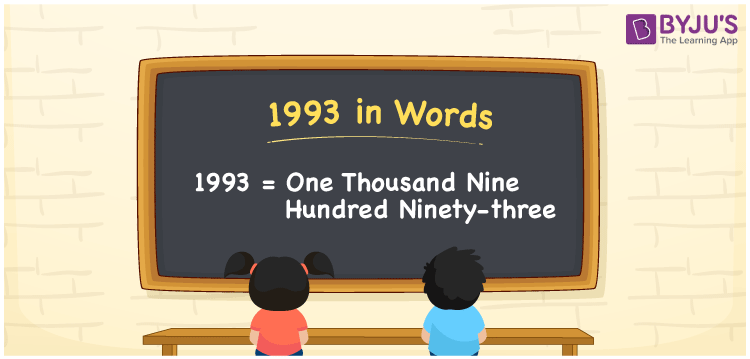# 1993 in Words

We can write the number 1993 in words as One Thousand Nine Hundred Ninety-Three. In Maths, we know that numbers are used to represent the value of something. For example, Rahul purchased a pair of shoes worth Rs. 1993, then you can say, “Rahul purchased a pair of shoes worth Rupees One Thousand Nine Hundred Ninety-Three”. 1993 is a cardinal number since it represents a certain amount. In this article, let us learn the conversion of the number 1993 into words with the help of a place value chart.

 1993 in Words One Thousand Nine Hundred Ninety-Three One Thousand Nine Hundred Ninety-Three in numerical form 1993

## 1993 in English Words

In Mathematics, generally, we write the numbers in words using the English alphabet. Hence, we can write the number 1993 in English as One Thousand Nine Hundred Ninety-Three.## How to Write 1993 in Words?

Place value chart is helpful in converting the number to word form. Here,1993 is a four-digit number. So, let us make a chart of 4 columns to represent the place value chart for the number 1993. This can be done as follows.

 Thousands Hundreds Tens Ones 1 9 9 3

Therefore, we can write the expanded form as:

1 x Thousand + 9 x Hundred + 9 x Ten + 3 x One

= 1 x 1000 + 9 x 100 + 9 x 10 + 3 x 1

= 1000 + 900 + 90 + 3

= 1993

= One Thousand Nine Hundred Ninety-Three

Hence, 1993 in words is written as One Thousand Nine Hundred Ninety-Three

Interesting way of writing 1993 in words

1 = One

19 = Nineteen

199 = One Hundred and Ninety-Nine

1993 = One Thousand Nine Hundred Ninety-Three

Thus, the word form of the number 1993 is One Thousand Nine Hundred Ninety-Three

1993 is a natural number that precedes 1994 and succeeds 1992

• 1993 in words – One Thousand Nine Hundred Ninety-Three
• Is 1993 an odd number? – Yes
• Is 1993 an even number? – No
• Is 1993 a perfect square number? – No
• Is 1993 a perfect cube number? – No
• Is 1993 a prime number? – Yes
• Is 1993 a composite number? – No

## Frequently Asked Questions on 1993 in Words

Q1

### What is 1993 in words?

1993 in words is One Thousand Nine Hundred Ninety-Three
Q2

### What is the value of 1993 + 2003?

1993 + 2003 = 3996. Hence, the value of 1993 + 2003 is 3996.
Q3

### 1993 is an even number. True or False.

False, 1993 is not an even number. It is an odd number.